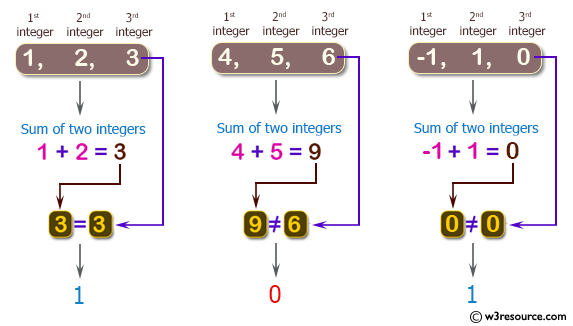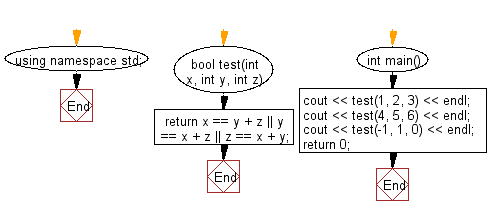﻿ C++ : Add two integers to get the third from three integers# C++ Exercises: Check if it is possible to add two integers to get the third integer from three given integers

## C++ Basic Algorithm: Exercise-44 with Solution

Write a C++ program to check if it is possible to add two integers to get the third integer from three given integers.

Sample Solution:

C++ Code :

``````#include <iostream>

using namespace std;

bool test(int x, int y, int z)
{
return x == y + z || y == x + z || z == x + y;
}

int main()
{
cout << test(1, 2, 3) << endl;
cout << test(4, 5, 6) << endl;
cout << test(-1, 1, 0) << endl;
return 0;
}
``````

Sample Output:

```1
0
1
```

Pictorial Presentation:Flowchart:C++ Code Editor:

Contribute your code and comments through Disqus.

What is the difficulty level of this exercise?

﻿